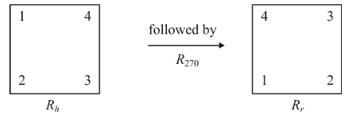Chapter 8.3, Problem 27ESMathematical Excursions (MindTap C...

4th Edition
Richard N. Aufmann + 3 others
ISBN: 9781305965584

Solutions

Chapter
SectionMathematical Excursions (MindTap C...

4th Edition
Richard N. Aufmann + 3 others
ISBN: 9781305965584
Textbook Problem

We can form another symmetry group by using rotations of a square rather than of a triangle. Start with a square with corners labeled 1 through 4, placed in a reference square.The current position of the inner square is labeled I . We can rotate the inner square clockwise 90 ° to give an element of the group, R 90 . Similarly, we can rotate the square 180 ° or 270 ° to obtain the elements R 180 and R 270 . In addition, we can rotate the square about any of the lines of symmetry shown below.As before, the operation is “followed by," denoted by Δ . Refer to this symmetry group of the square.Find R h Δ R 270 .

To determine

Refer to symmetry group of square and find RhΔR270

Explanation

Calculation:

Considering the symmetry group of square, we need to find RhΔR270

Let the original square I as follows

Let us rotate the square about the horizontal axis and then rotate through 270

Still sussing out bartleby?

Check out a sample textbook solution.

See a sample solution

The Solution to Your Study Problems

Bartleby provides explanations to thousands of textbook problems written by our experts, many with advanced degrees!

Get Started

In Problems 1-6 simplify each fraction. 3.

Mathematical Applications for the Management, Life, and Social Sciences

Differentiate. f() = cos sin

Single Variable Calculus: Early Transcendentals, Volume I

Solve the equations in Exercises 112 for x (mentally, if possible). ax+b=c(a0)

Finite Mathematics and Applied Calculus (MindTap Course List)